Courses

# Circles - General Geometry Mathematics Notes | EduRev

## Mathematics : Circles - General Geometry Mathematics Notes | EduRev

The document Circles - General Geometry Mathematics Notes | EduRev is a part of the Mathematics Course Additional Topics for IIT JAM Mathematics.
All you need of Mathematics at this link: Mathematics

A circle is all points in the same plane that lie at an equal distance from a center point. The circle is only composed of the points on the border. You could think of a circle as a hula hoop. It's only the points on the border that are the circle. The points within the hula hoop are not part of the circle and are called interior points.
The distance between the midpoint and the circle border is called the radius. A line segment that has the endpoints on the circle and passes through the midpoint is called the diameter. The diameter is twice the size of the radius. A line segment that has its endpoints on the circular border but does not pass through the midpoint is called a chord.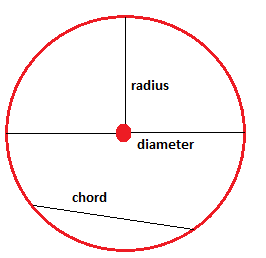The distance around the circle is called the circumference, C, and could be determined either by using the radius, r, or the diameter, d: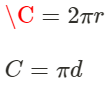A circle is the same as 360°. You can divide a circle into smaller portions. A part of a circle is called an arc and an arc is named according to its angle. Arcs are divided into minor arcs (0° < v < 180°), major arcs (180° < v < 360°) and semicircles (v = 180°).
The length of an arc, l, is determined by plugging the degree measure of the Arc, v, and the circumference of the whole circle, C, into the following formula: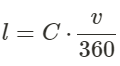When diameters intersect at the central of the circle they form central angles. Like when you cut a cake you begin your pieces in the middle.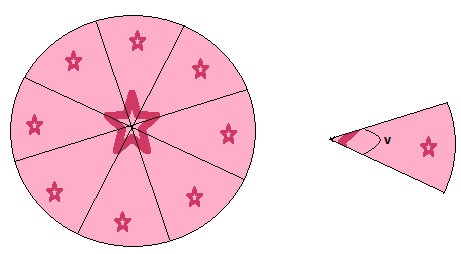Example
As in the cake above we divide our circle into 8 pieces with the same angle. The circumference of the circle is 20 length units. Determine the length of the arc of each piece.
First we need to find the angle for each piece, since we know that a full circle is 360° we can easily tell that each piece has an angle of 360/8 = 45°. We plug these values into our formula for the length of arcs: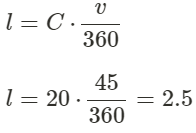Hence the length of our arcs are 2.5 length units. We could even easier have told this by simply diving the circumference by the number of same size pieces: 20/8 = 2.5

Inscribed angles and polygons
An inscribed angle is an angle that has its vertex on the circle and the rays of the angle are cords of the circle.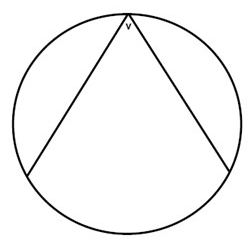If we have one angle that is inscribed in a circle and another that has the same starting points but its vertex is in the center of the circle then the second angle is twice the angle that is inscribed: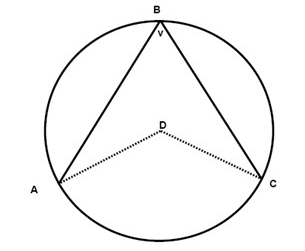Just as an angle could be inscribed into a circle a polygon could be inscribed into a circle as well: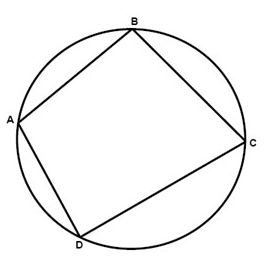If a quadrilateral (as in the figure above) is inscribed in a circle, then its opposite angles are supplementary:
∠A and ∠C are supplementary
∠B and ∠D are supplementary

A line that intersects a circle in exactly one point is called a tangent and the point where the intersection occurs is called the point of tangency. The tangent is always perpendicular to the radius drawn to the point of tangency.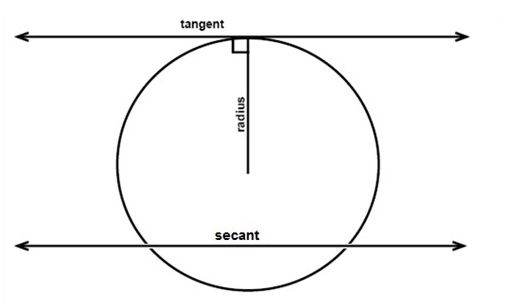A secant is a line that intersects a circle in exactly two points.
When a tangent and a secant, two secants, or two tangents intersect outside a circle then the measure of the angle formed is one-half the positive difference of the measures of the intercepted arcs.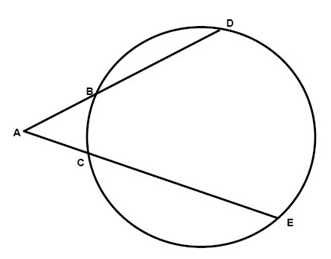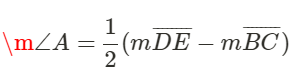When two chords intersect inside a circle, then the measures of the segments of each chord multiplied with each other is equal to the product from the other chord: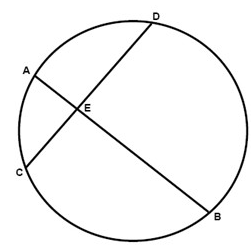AB⋅EB = CE⋅ED
If two secants are drawn to a circle from one exterior point, then the product of the external segment and the total length of each secant are equal: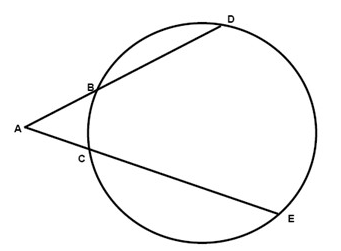If one secant and one tangent are drawn to a circle from one exterior point, then the square of the length of the tangent is equal to the product of the external secant segment and the total length of the secant: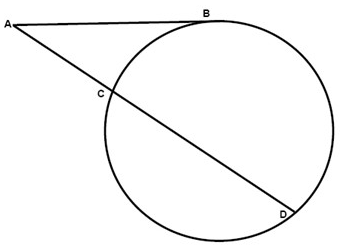If we have a circle drawn in a coordinate plane, with the center in (a,b) and the radius r then we could always describe the circle with the following equation:
(x−a)2+(y−b)2 = r2

Offer running on EduRev: Apply code STAYHOME200 to get INR 200 off on our premium plan EduRev Infinity!

40 docs

,

,

,

,

,

,

,

,

,

,

,

,

,

,

,

,

,

,

,

,

,

;